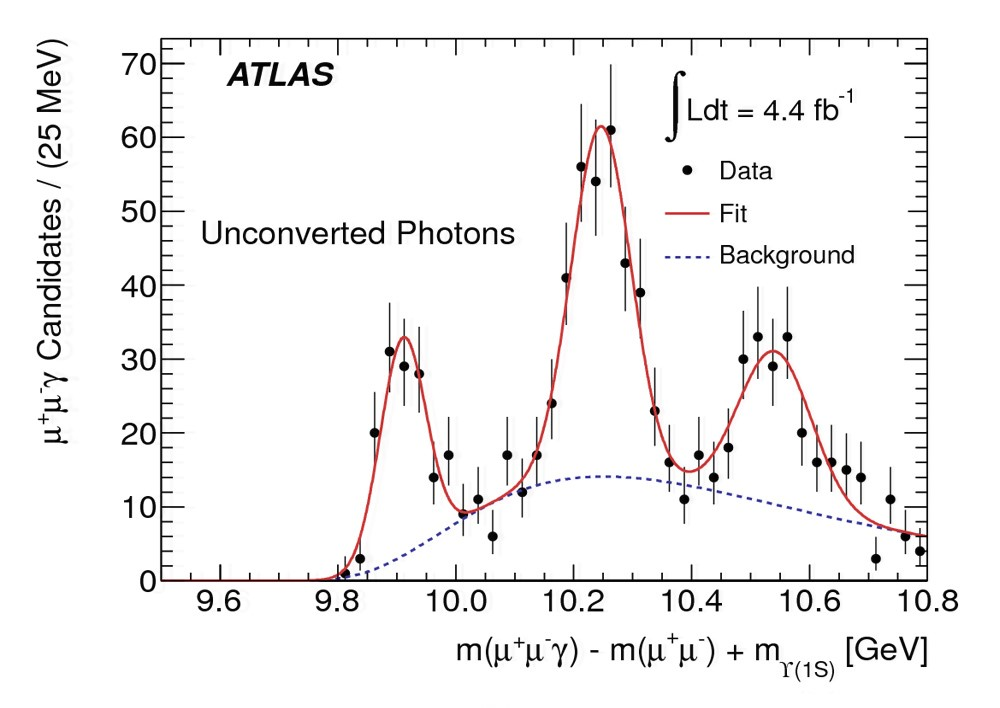The spectrum of the χb states: the leftmost peak is the χb(1P), the middle one the χb(2P), and the rightmost the new χb(3P). The upper plot shows the spectrum for decays involving unconverted photons, while th lower plot shows the spectra for decays involving converted photons. In the lower plot, the upper (red) curve shows the spectrum for χb decays to Υ(1S), while the lower (brown) curve shows the spectrum for decays to Υ(2S). (Only the χb(3P) peak appears distinctly in the lower spectrum because it is the only χb state with decays involving enough energy to be detected in this study.)

The ATLAS collaboration has announced the discovery of the χb(3P), which is a bound state of a bottom quark and bottom antiquark (bbar). Bound states of a heavy quark and its antiquark are collectively called quarkonium. They are the QCD (Quantum Chromodynamics or strong force) analogues of the hydrogen atom, with each new particle corresponding to a different energy level. For bbar states, the P states are called χb (chi b) and the S states Υ (upsilon). Like the hydrogen atom, we can observe transitions between these states through emission of a photon γ, and this new state was discovered through the radiative transitions χb(3P) → Υ(1S) + γ and transitions
χb(3P) → Υ(2S) + γ followed by the decay of the upsilon (itself a lighter quarkonium state) to two muons.

The figure shows the spectrum of the χ states: the leftmost peak is the χb(1P), the middle one the χb(2P), and the rightmost the new χb(3P). The photons are detected either by the electromagnetic calorimeter (unconverted) or by the ATLAS tracking detectors if they have interacted with material and converted to an e+e pair.

As we learned about the hydrogen atom by studying its energy levels, we can learn about the forces binding quarks together by studying the energy levels of quarkonium states. The χb(3P) is just at the very limit of being bound, so the quark and antiquark are about as far apart from each other as they can possibly be. One surprise is that the χb(3P) is slightly heavier than predicted, meaning the quark anti-quark pair is a little more loosely bound than expected.

Also in analogy with atomic physics, the visible peaks contain internal structure due to hyperfine splitting among states of different angular momentum. These could be resolved with future data samples.

Normally, a new particle is discovered in one or at most two channels, and the first discovery is at the very edge of statistical significance. χb(3P) is different: it's seen in three different channels, and the peaks are unmistakable. The outstanding LHC performance is responsible for this by delivering so many collisions in such a short time.

A publication has been submitted to Physical Review Letters.